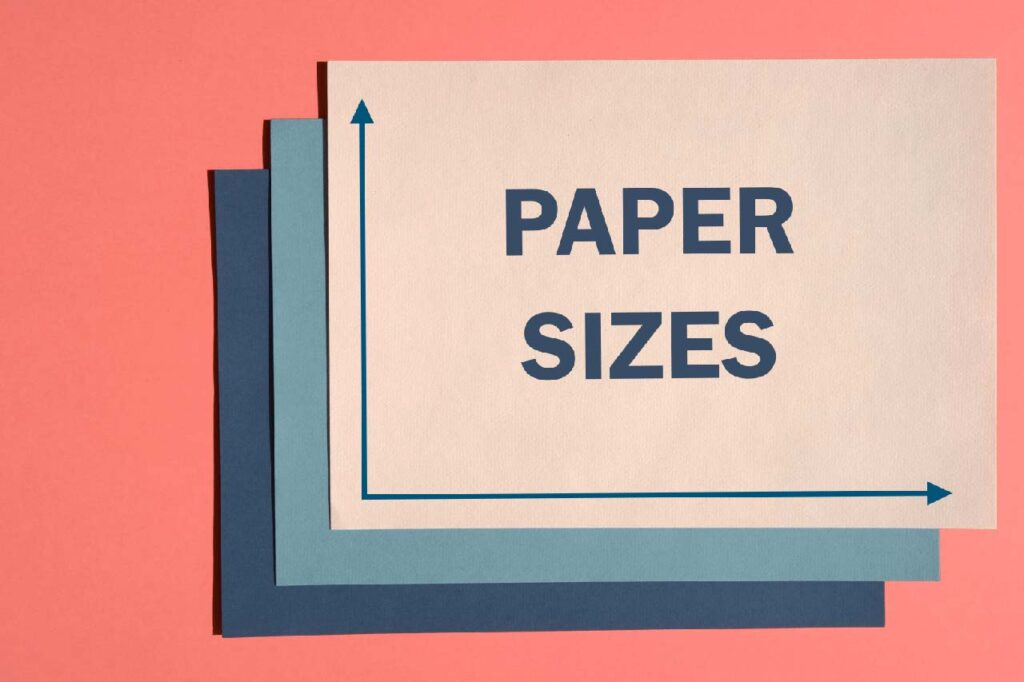# Paper sizesWe usually take prints in an A4 size paper. The size of this paper is 210 x 297mm or 8.3 x 11.7 in. There are other paper sizes also.

The A paper size system was formally adopted in Europe in the 19th century and has since spread around the world. It is now used in nearly every country apart from the USA and Canada. A4 is the most common standard business letter size used in English speaking countries. This is the ISO 216 paper size system. This system is an international standard that is used in many places in the world in copy and print devices that defines paper sizes. Based on the metric system, the ISO paper size system uses a height-to-width ratio with an aspect ratio of 1 to 1.414 (The square root of 2). If a sheet is cut into two, the resulting halves are the same proportions as the original. The United States and Canada do not widely use the ISO standard paper sizes. Instead, the United States uses the Letter, Legal and Executive system.

Given below is a list of various paper sizes used in India.

• A0 = 841 x 1189 mm 33.1 x 46.8 in
• A1 = 594 x 841 mm 23.4 x 33.1 in
• A2 = 420 x 594 mm 16.5 x 23.4 in
• A3 = 297 x 420 mm 11.7 x 16.5 in
• A4 = 210 x 297 mm 8.3 x 11.7 in
• A5 = 148 x 210 mm 5.8 x 8.3 in
• A6 = 105 x 148 mm 4.1 x 5.8 in
• A7 = 74 x 105 mm 2.9 x 4.1 in
• A8 = 52 x 74 mm 2.0 x 2.9 in
• A9 = 37 x 52 mm 1.5 x 2.0 in
• A10 = 26 x 37 mm 1.0 x 1.5 in

To obtain paper sizes in centimetres, convert mm values to cm by dividing by 10 and in feet by dividing inch values by 12.

0 Shares